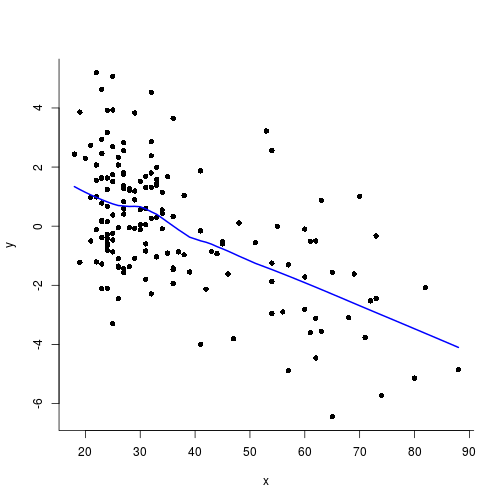# Introduction to mixed-effects modelling

Christophe Pallier
2017-05-29

### Plan

• Some generalities about statistical inference
• Classic regression and its implementation in R
• Random effects and mixed-effects models
• Examples of mixed-effects analyses with R

### Meaning of "X has an effect on Y" mean

What does it mean, from a statistical point of view, to say that the variable $$X$$ has an effect on the variable $$Y$$?

$$Y$$ is influenced by $$X$$ if the *distribution of $$Y$$ change when $$X$$ takes different values.

### Example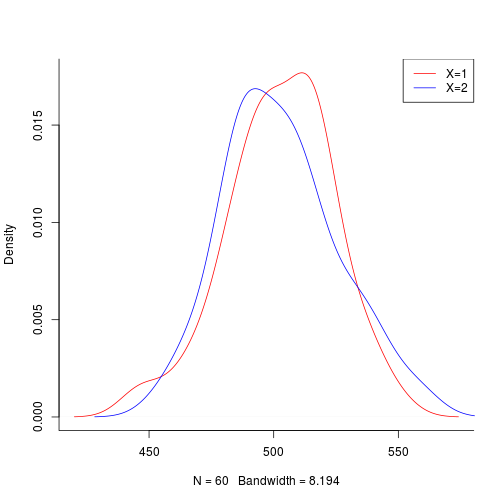As the two distributions differ, we can state that “$$X$$ has an effect on $$Y$$”“ (not implying causality).

Remark: One is often only interested in whether the means of the distributions differ, e.g.: $E(Y|X=1) \stackrel{?}{=} E(Y|X=2)$

### Sampling

In real life, we rarely have access to the data of the whole population but only to random samples, that is, to a finite set of observations $$(y_i)$$.

The distributions of the samples can (and often will) differ from one another because of sampling variability, that is, the fact that different individuals compose each sample.

For example, the mean heights of two groups of 20 boys will not be exactly the same.

### Inference

To make statements about a population property from observed samples, we need statistical inference.

1. hypothesis testing (e.g. does the mean of $$Y$$ (e.g. height) varies with $$X$$ (e.g. nationality)?)
2. estimation (e.g. what is a confidence interval for the average height of French people?)
3. prediction (e.g. what is the most probable height of a 18 year old German boy?)

### Classical Simple Regression

• One predictor variable $$X$$
• One dependent variable $$Y$$ (continuous).

The question of interest is whether $$E(Y|X)$$ is constant or not:

$\cal{H}_0: \quad \rm E(Y|X=x) = \beta, \quad x \ \mbox{varying}$

One alternative hypothesis is that the average of $$Y$$ depends linearily on $$X$$ :

$\cal{H}_{lin}: \quad \rm E(Y|X=x) = \alpha x + \beta$

### Graphical Representation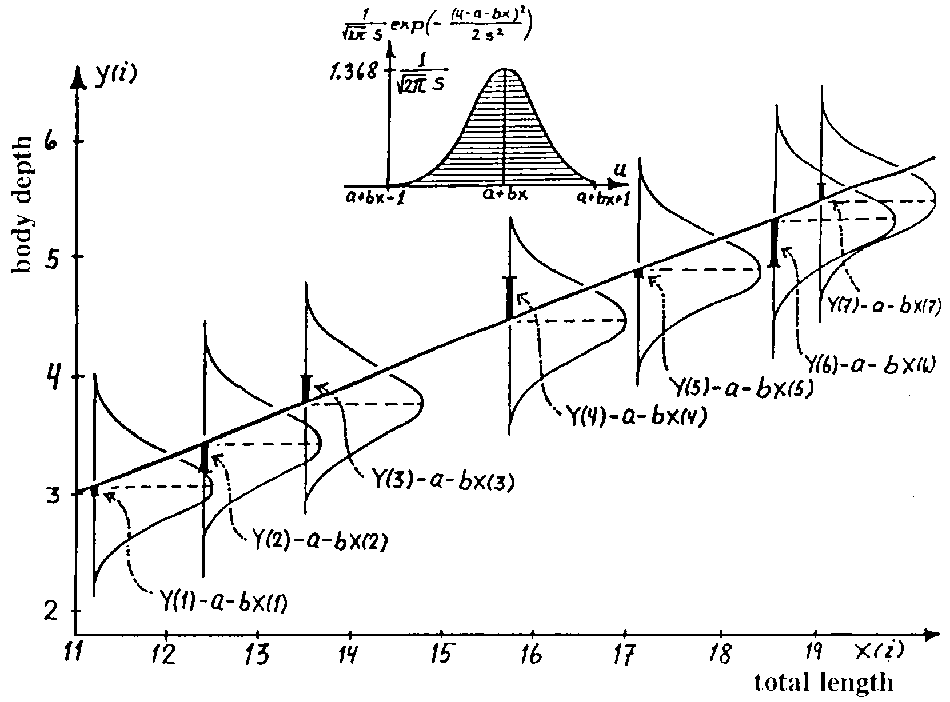### Example

Effect of age on tot, a biomarker of kidney functioning.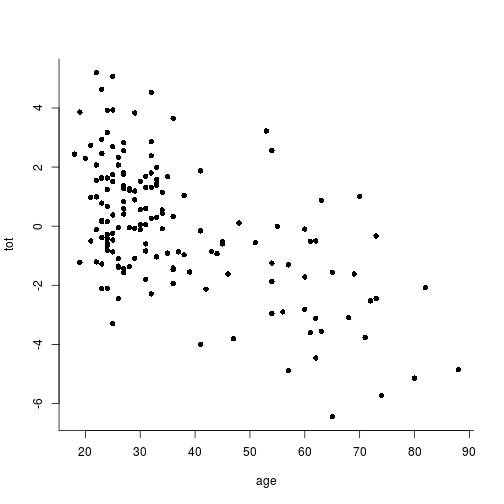### Ordinary Least Squares solution to Regression

The OLS method searches among all linear models one that minimizes the Euclidian distance between the model's predictions and the data ($$\sum (y_i - \hat{y}_i)^2$$).

In R, this is accomplished by the fit function:

x = age
y = tot
fit = lm(y ~ x)
coef(fit)

(Intercept)           x
2.86002680 -0.07858842


These coefficients estimate the population parameters $$\beta$$ and $$\alpha$$.

### Prediction

The equation $$\alpha.x+\beta$$ can be used to compute the expected value of $$Y$$ for a given value $$x$$ of $$X$$

plot(y ~ x, bty='l', pch=16)
abline(fit, col=2, lwd=2)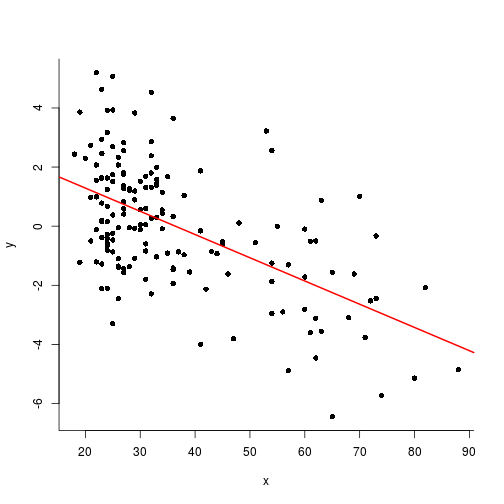### Hyothesis testing in simple regression

To draw any inference from these coefficients to the population parameters, for example to know their precision, you need an additional hypothesis.

The simplest one is the the errors terms are statistically independent and each is distributed following a normal distribution with a standard deviation $$\sigma$$ :

$y_i = \alpha . x_i + \beta + \epsilon_i , \quad \epsilon \sim \cal{N}(0,\sigma^2)$

### Testing the hypothesis of a non-null slope

The p-value for the hypothesis that $$X$$ has no (linear) effect on $$Y$$ can be obtained calling the function anova with the linear model object as a parameter.

anova(fit)

Analysis of Variance Table

Response: y
Df Sum Sq Mean Sq F value    Pr(>F)
x           1 244.29 244.288  75.312 5.182e-15 ***
Residuals 155 502.77   3.244
---
Signif. codes:  0 '***' 0.001 '**' 0.01 '*' 0.05 '.' 0.1 ' ' 1


### Estimates and standard errors of coefficients

They can be displayed with the function summary, allowing to construct confidence intervals for them:

summary(fit)


Call:
lm(formula = y ~ x)

Residuals:
Min      1Q  Median      3Q     Max
-4.2018 -1.3451  0.0765  1.0719  4.5252

Coefficients:
Estimate Std. Error t value Pr(>|t|)
(Intercept)  2.860027   0.359565   7.954 3.53e-13 ***
x           -0.078588   0.009056  -8.678 5.18e-15 ***
---
Signif. codes:  0 '***' 0.001 '**' 0.01 '*' 0.05 '.' 0.1 ' ' 1

Residual standard error: 1.801 on 155 degrees of freedom
Multiple R-squared:  0.327, Adjusted R-squared:  0.3227
F-statistic: 75.31 on 1 and 155 DF,  p-value: 5.182e-15


### Remark 1: X can be binary

The regression regression of $$Y$$ on $$X$$ is strictly equivalent to a Student T test.

x = rep(1:2, 20)
y = x + rnorm(40)

m1 <- lm(y ~ x)
plot(y ~ x, pch=16, xlim=c(.5, 2.5))
abline(m1)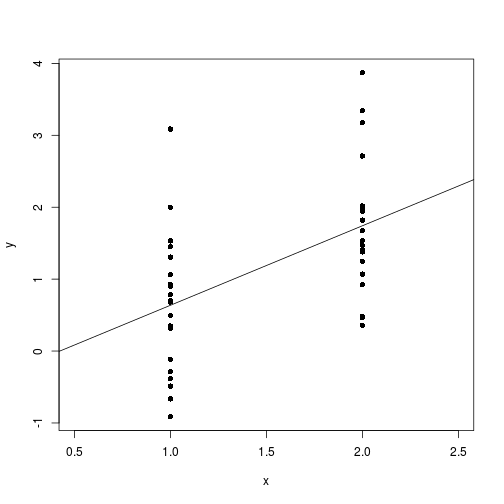### Case of binary X

summary(m1)


Call:
lm(formula = y ~ x)

Residuals:
Min       1Q   Median       3Q      Max
-1.55111 -0.69204 -0.01078  0.32311  2.45041

Coefficients:
Estimate Std. Error t value Pr(>|t|)
(Intercept)  -0.4690     0.4822  -0.973 0.336914
x             1.1057     0.3050   3.626 0.000842 ***
---
Signif. codes:  0 '***' 0.001 '**' 0.01 '*' 0.05 '.' 0.1 ' ' 1

Residual standard error: 0.9644 on 38 degrees of freedom
Multiple R-squared:  0.257, Adjusted R-squared:  0.2375
F-statistic: 13.15 on 1 and 38 DF,  p-value: 0.0008421

t.test(y ~ x, var.equal=T)


Two Sample t-test

data:  y by x
t = -3.6258, df = 38, p-value = 0.0008421
alternative hypothesis: true difference in means is not equal to 0
95 percent confidence interval:
-1.7230634 -0.4883563
sample estimates:
mean in group 1 mean in group 2
0.6367533       1.7424631


### Remark 2: testing for non linear effect of X

x = age
y = tot
m1 <- lm(y ~ x)
summary(m2 <- lm(y ~ poly(x, 2))) # in '*linear model*', '*linear*' stands for a the relationship between the parameters and Y, not the predictors and Y


Call:
lm(formula = y ~ poly(x, 2))

Residuals:
Min      1Q  Median      3Q     Max
-4.2024 -1.3492  0.0449  1.0755  4.4781

Coefficients:
Estimate Std. Error t value Pr(>|t|)
(Intercept) -1.911e-04  1.442e-01  -0.001    0.999
poly(x, 2)1 -1.563e+01  1.806e+00  -8.652 6.26e-15 ***
poly(x, 2)2 -4.758e-01  1.806e+00  -0.263    0.793
---
Signif. codes:  0 '***' 0.001 '**' 0.01 '*' 0.05 '.' 0.1 ' ' 1

Residual standard error: 1.806 on 154 degrees of freedom
Multiple R-squared:  0.3273,    Adjusted R-squared:  0.3186
F-statistic: 37.46 on 2 and 154 DF,  p-value: 5.522e-14


### Remark 2: testing for non linear effect of X

plot(x, y)
lines(x, fitted(m1), col=2) # linear
lines(x, fitted(m2), col=3) # polynomial deg 2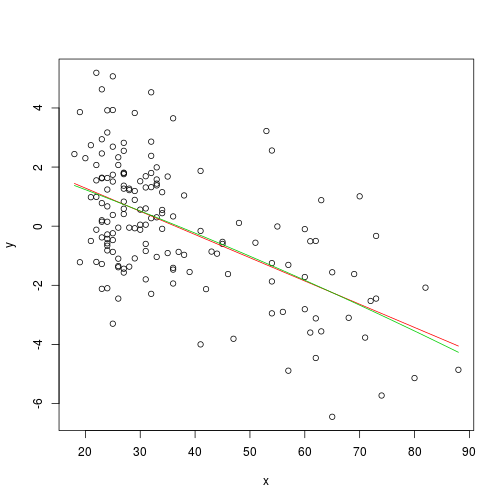### Remark 3: Non-parametric analyses as an alternative

plot(y ~ x, bty='l', pch=16)
lines(lowess(x, y), col='blue', lwd=2)